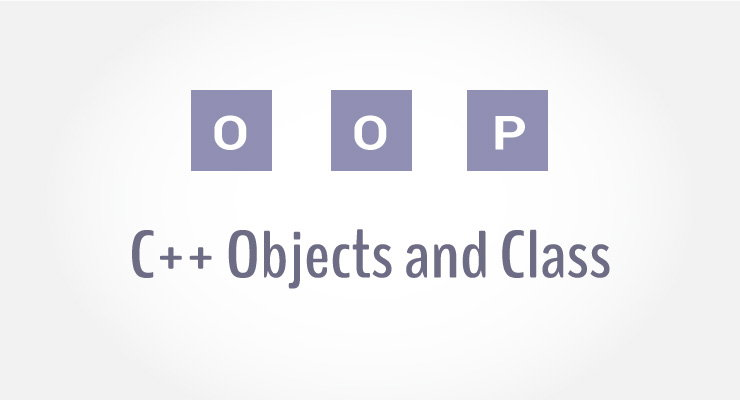# C++ Classes and Objects

In this article, you will learn to work with objects and classes in C++ programming.C++ is a multi-paradigm programming language. Meaning, it supports different programming styles.

One of the popular ways to solve a programming problem is by creating objects, known as object-oriented style of programming.

C++ supports object-oriented (OO) style of programming which allows you to divide complex problems into smaller sets by creating objects.

Object is simply a collection of data and functions that act on those data.

## C++ Class

Before you create an object in C++, you need to define a class.

A class is a blueprint for the object.

We can think of class as a sketch (prototype) of a house. It contains all the details about the floors, doors, windows etc. Based on these descriptions we build the house. House is the object.

As, many houses can be made from the same description, we can create many objects from a class.

### How to define a class in C++?

A class is defined in C++ using keyword `class` followed by the name of class.

The body of class is defined inside the curly brackets and terminated by a semicolon at the end.

```class className
{
// some data
// some functions
};```

### Example: Class in C++

``````class Test
{
private:
int data1;
float data2;

public:
void function1()
{   data1 = 2;  }

float function2()
{
data2 = 3.5;
return data2;
}
};``````

Here, we defined a class named `Test`.

This class has two data members: data1 and data2 and two member functions: function1() and function2().

### Keywords: private and public

You may have noticed two keywords: private and public in the above example.

The private keyword makes data and functions private. Private data and functions can be accessed only from inside the same class.

The public keyword makes data and functions public. Public data and functions can be accessed out of the class.

Here, data1 and data2 are private members where as function1() and function2() are public members.

If you try to access private data from outside of the class, compiler throws error. This feature in OOP is known as data hiding.

## C++ Objects

When class is defined, only the specification for the object is defined; no memory or storage is allocated.

To use the data and access functions defined in the class, you need to create objects.

### Syntax to Define Object in C++

`className objectVariableName;`

You can create objects of Test class (defined in above example) as follows:

``````
class Test
{
private:
int data1;
float data2;

public:
void function1()
{   data1 = 2;  }

float function2()
{
data2 = 3.5;
return data2;
}
};

int main()
{
Test o1, o2;
}
``````

Here, two objects o1 and o2 of Test class are created.

In the above class Test, data1 and data2 are data members and function1() and function2() are member functions.

### How to access data member and member function in C++?

You can access the data members and member functions by using a . (dot) operator. For example,

`o2.function1();`

This will call the function1() function inside the Test class for objects o2.

Similarly, the data member can be accessed as:

`o1.data2 = 5.5;`

It is important to note that, the private members can be accessed only from inside the class.

So, you can use `o2.function1();` from any function or class in the above example. However, the code `o1.data2 = 5.5;` should always be inside the class Test.

### Example: Object and Class in C++ Programming

``````// Program to illustrate the working of objects and class in C++ Programming
#include <iostream>
using namespace std;

class Test
{
private:
int data1;
float data2;

public:

void insertIntegerData(int d)
{
data1 = d;
cout << "Number: " << data1;
}

float insertFloatData()
{
cout << "\nEnter data: ";
cin >> data2;
return data2;
}
};

int main()
{
Test o1, o2;
float secondDataOfObject2;

o1.insertIntegerData(12);
secondDataOfObject2 = o2.insertFloatData();

cout << "You entered " << secondDataOfObject2;
return 0;
}
``````

Output

```Number: 12
Enter data: 23.3
You entered 23.3```

In this program, two data members data1 and data2 and two member functions `insertIntegerData()` and `insertFloatData()` are defined under Test class.

Two objects o1 and o2 of the same class are declared.

The `insertIntegerData()` function is called for the o1 object using:

`o1.insertIntegerData(12);`

This sets the value of data1 for object o1 to 12.

Then, the `insertFloatData()` function for object o2 is called and the return value from the function is stored in variable secondDataOfObject2 using:

`secondDataOfObject2 = o2.insertFloatData();`

In this program, data2 of o1 and data1 of o2 are not used and contains garbage value.

You should also check these topics to learn more on objects and classes: